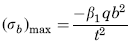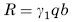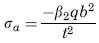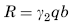Related Resources: calculators

### Two Edges Fixed Two Edges Free Loading Uniform over Entire Plate Equation and Calculator

Flat Rectangular Plate, Two Edges Fixed, Two Edges Free Loading Uniform over Entire Plate Equation and Calculator.

Per. Roarks Formulas for Stress and Strain for flat plates with straight boundaries and constant thickness

Flat Rectangular plate, Two Edges Fixed, Two Edges Free Loading Uniform over Entire Plate.

 Rectangular plate; Two Edges Fixed Two Edges FreeAt x = 0, z = 0
Stress and Reaction ForceReaction ForceStress and Reaction Force at x = 0, z = b if a > b/2 or a = 0.8b if ≤ b/2Reaction ForceWhere used:
E = Modulus of Elasticity (lbs/in2)
q = Total load or force to Plate (lbs/in2)
v = Poisson’s ratio (assumed to be 0.3)
t = plate thickness, (in)
a = plate length, (in)
b = plate width, (in)
σb = stress, (lbs/in2)
R = Reaction Force (lbs/in)
β1,2 = Constant From Table A
γ1,2 = Constant From Table A

Table A

 a/b 0.125 0.25 0.375 0.5 0.75 1 β1 0.05 0.182 0.353 0.631 1.246 1.769 β2 0.047 0.188 0.398 0.632 1.186 1.769 γ1 0.312 0.572 0.671 0.874 1.129 1.183 γ2 0.127 0.264 0.413 0.557 0.829 1.183

Reference:

Roarks Formulas for Stress and Strain, 7th Edition